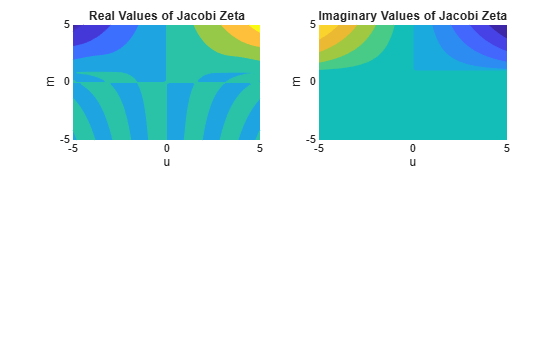# jacobiZeta

Jacobi zeta function

## Syntax

``jacobiZeta(u,m)``

## Description

example

````jacobiZeta(u,m)` returns the Jacobi Zeta Function of `u` and `m`. If `u` or `m` is an array, then `jacobiZeta` acts element-wise.```

## Examples

collapse all

`jacobiZeta(2,1)`
```ans = 0.9640```

Call `jacobiZeta` on array inputs. `jacobiZeta` acts element-wise when `u` or `m` is an array.

`jacobiZeta([2 1 -3],[1 2 3])`
```ans = 0.9640 + 0.0000i 0.5890 - 0.4569i -2.3239 + 1.9847i```

Convert numeric input to symbolic form using `sym`, and find the Jacobi zeta function. For symbolic input where `u = 0` or `m = 0` or `1`, `jacobiZeta` returns exact symbolic output.

`jacobiZeta(sym(2),sym(1))`
```ans = tanh(2)```

Show that for other values of `u` or `m`, `jacobiZeta` returns an unevaluated function call.

`jacobiZeta(sym(2),sym(3))`
```ans = jacobiZeta(2, 3)```

For symbolic variables or expressions, `jacobiZeta` returns the unevaluated function call.

```syms x y f = jacobiZeta(x,y)```
```f = jacobiZeta(x, y)```

Substitute values for the variables by using `subs`, and convert values to double by using `double`.

`f = subs(f, [x y], [3 5])`
```f = jacobiZeta(3, 5)```
`fVal = double(f)`
```fVal = 4.0986 - 3.0018i```

Calculate `f` to arbitrary precision using `vpa`.

`fVal = vpa(f)`
```fVal = 4.0986033838332279126523721581432 - 3.0017792319714320747021938869936i```

Plot real and imaginary values of the Jacobi zeta function using `fcontour`. Set `u` on the x-axis and `m` on the y-axis by using the symbolic function `f` with the variable order `(u,m)`. Fill plot contours by setting `Fill` to `on`.

```syms f(u,m) f(u,m) = jacobiZeta(u,m); subplot(2,2,1) fcontour(real(f),'Fill','on') title('Real Values of Jacobi Zeta') xlabel('u') ylabel('m') subplot(2,2,2) fcontour(imag(f),'Fill','on') title('Imaginary Values of Jacobi Zeta') xlabel('u') ylabel('m')```## Input Arguments

collapse all

Input, specified as a number, vector, matrix, or multidimensional array, or a symbolic number, variable, vector, matrix, multidimensional array, function, or expression.

Input, specified as a number, vector, matrix, or multidimensional array, or a symbolic number, variable, vector, matrix, multidimensional array, function, or expression.

collapse all

### Jacobi Zeta Function

The Jacobi zeta function `jacobiZeta(u,m)` is defined as

`$Z\left(u,m\right)=E\left(\phi ,m\right)-\frac{E\left(m\right)}{K\left(m\right)}F\left(\phi ,m\right).$`

The definitions of the terms in above equation are:

The argument u is related to φ by the relations u = F(φ | m) and am(u, m) = φ, where am(u, m) is the Jacobi's amplitude function.

 Olver, F. W. J., A. B. Olde Daalhuis, D. W. Lozier, B. I. Schneider, R. F. Boisvert, C. W. Clark, B. R. Miller, B. V. Saunders, H. S. Cohl, and M. A. McClain, eds., Chapter 22. Jacobian Elliptic Functions, NIST Digital Library of Mathematical Functions, Release 1.0.26 of 2020-03-15.